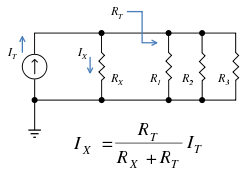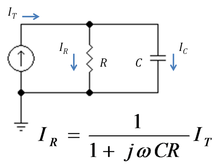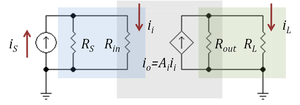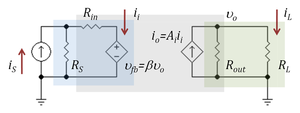# Current divider

﻿
Current dividerFigure 1: Schematic of an electrical circuit illustrating current division. Notation RT. refers to the total resistance of the circuit to the right of resistor RX.

In electronics, a current divider is a simple linear circuit that produces an output current (IX) that is a fraction of its input current (IT). Current division refers to the splitting of current between the branches of the divider. The currents in the various branches of such a circuit will always divide in such a way as to minimize the total energy expended.

The formula describing a current divider is similar in form to that for the voltage divider. However, the ratio describing current division places the impedance of the unconsidered branches in the numerator, unlike voltage division where the considered impedance is in the numerator. This is because in current dividers, total energy expended is minimized, resulting in currents that go through paths of least impedance, therefore the inverse relationship with impedance. On the other hand, voltage divider is used to satisfy Kirchoff's Voltage Law. The voltage around a loop must sum up to zero, so the voltage drops must be divided evenly in a direct relationship with the impedance.

To be specific, if two or more impedances are in parallel, the current that enters the combination will be split between them in inverse proportion to their impedances (according to Ohm's law). It also follows that if the impedances have the same value the current is split equally.

## Resistive divider

A general formula for the current IX in a resistor RX that is in parallel with a combination of other resistors of total resistance RT is (see Figure 1):$I_X = \frac{R_T}{R_X+R_T}I_T \$

where IT is the total current entering the combined network of RX in parallel with RT. Notice that when RT is composed of a parallel combination of resistors, say R1, R2, ... etc., then the reciprocal of each resistor must be added to find the total resistance RT:$\frac {1}{R_T} = \frac {1} {R_1} + \frac {1} {R_2} + \frac {1}{R_3} + ... \ .$

## General case

Although the resistive divider is most common, the current divider may be made of frequency dependent impedances. In the general case the current IX is given by:$I_X = \frac{Z_T} {Z_X+Z_T}I_T \ ,$

Instead of using impedances, the current divider rule can be applied just like the voltage divider rule if admittance (the inverse of impedance) is used.$I_X = \frac{Y_X} {Y_{Total}}I_T$

Take care to note that YTotal is a straightforward addition, not the sum of the inverses inverted (as you would do for a standard parallel resistive network). For Figure 1, the current IX would be$I_X = \frac{Y_X} {Y_{Total}}I_T = \frac{\frac{1}{R_X}} {\frac{1}{R_X} + \frac{1}{R_1} + \frac{1}{R_2} + \frac{1}{R_3}}I_T$

### Example: RC combinationFigure 2: A low pass RC current divider

Figure 2 shows a simple current divider made up of a capacitor and a resistor. Using the formula above, the current in the resistor is given by:$I_R = \frac {\frac{1}{j \omega C}} {R + \frac{1}{j \omega C} }I_T$$= \frac {1} {1+j \omega CR} I_T \ ,$

where ZC = 1/(jωC) is the impedance of the capacitor.

The product τ = CR is known as the time constant of the circuit, and the frequency for which ωCR = 1 is called the corner frequency of the circuit. Because the capacitor has zero impedance at high frequencies and infinite impedance at low frequencies, the current in the resistor remains at its DC value IT for frequencies up to the corner frequency, whereupon it drops toward zero for higher frequencies as the capacitor effectively short-circuits the resistor. In other words, the current divider is a low pass filter for current in the resistor.Figure 3: A current amplifier (gray box) driven by a Norton source (iS, RS) and with a resistor load RL. Current divider in blue box at input (RS,Rin) reduces the current gain, as does the current divider in green box at the output (Rout,RL)

The gain of an amplifier generally depends on its source and load terminations. Current amplifiers and transconductance amplifiers are characterized by a short-circuit output condition, and current amplifiers and transresistance amplifiers are characterized using ideal infinite impedance current sources. When an amplifier is terminated by a finite, non-zero termination, and/or driven by a non-ideal source, the effective gain is reduced due to the loading effect at the output and/or the input, which can be understood in terms of current division.

Figure 3 shows a current amplifier example. The amplifier (gray box) has input resistance Rin and output resistance Rout and an ideal current gain Ai. With an ideal current driver (infinite Norton resistance) all the source current iS becomes input current to the amplifier. However, for a Norton driver a current divider is formed at the input that reduces the input current to$i_{i} = \frac {R_S} {R_S+R_{in}} i_S \ ,$

which clearly is less than iS. Likewise, for a short circuit at the output, the amplifier delivers an output current io = Ai ii to the short-circuit. However, when the load is a non-zero resistor RL, the current delivered to the load is reduced by current division to the value:$i_L = \frac {R_{out}} {R_{out}+R_{L}} A_i i_{i} \ .$

Combining these results, the ideal current gain Ai realized with an ideal driver and a short-circuit load is reduced to the loaded gain Aloaded:$A_{loaded} =\frac {i_L} {i_S} = \frac {R_S} {R_S+R_{in}}$$\frac {R_{out}} {R_{out}+R_{L}} A_i \ .$

### Unilateral versus bilateral amplifiersFigure 4: Current amplifier as a bilateral two-port network; feedback through dependent voltage source of gain β V/V

Figure 3 and the associated discussion refers to a unilateral amplifier. In a more general case where the amplifier is represented by a two port, the input resistance of the amplifier depends on its load, and the output resistance on the source impedance. The loading factors in these cases must employ the true amplifier impedances including these bilateral effects. For example, taking the unilateral current amplifier of Figure 3, the corresponding bilateral two-port network is shown in Figure 4 based upon h-parameters. Carrying out the analysis for this circuit, the current gain with feedback Afb is found to be$A_{fb} = \frac {i_L}{i_S} = \frac {A_{loaded}} {1+ {\beta}(R_L/R_S) A_{loaded}} \ .$

That is, the ideal current gain Ai is reduced not only by the loading factors, but due to the bilateral nature of the two-port by an additional factor ( 1 + β (RL / RS ) Aloaded ), which is typical of negative feedback amplifier circuits. The factor β (RL / RS ) is the current feedback provided by the voltage feedback source of voltage gain β V/V. For instance, for an ideal current source with RS = ∞ Ω, the voltage feedback has no influence, and for RL = 0 Ω, there is zero load voltage, again disabling the feedback.

## References and notes

1. ^ The h-parameter two port is the only two-port among the four standard choices that has a current-controlled current source on the output side.
2. ^ Often called the improvement factor or the desensitivity factor.

Wikimedia Foundation. 2010.

### Look at other dictionaries:

• Current-to-voltage converter — In electronics, a transimpedance amplifier is an amplifier that converts current to voltage. Its input ideally has zero impedance, and the input signal is a current. Its output may have low impedance, or in high frequency applications, may be… …   Wikipedia

• Voltage divider — In electronics, a voltage divider (also known as a potential divider) is a simple linear circuit that produces an output voltage ( V out) that is a fraction of its input voltage ( V in). Voltage division refers to the partitioning of a voltage… …   Wikipedia

• Voltage-to-current converter — Introduction For a variety of reasons, in low voltage electronics, voltage is a more frequently used data carrier. Thus electronic devices tend to be labeled with voltage inputs and outputs. However some devices are labeled in terms of current… …   Wikipedia

• Hall effect — For the Colombian band, see The Hall Effect (band). The Hall effect is the production of a voltage difference (the Hall voltage) across an electrical conductor, transverse to an electric current in the conductor and a magnetic field perpendicular …   Wikipedia

• Electrical resistance — is a ratio of the degree to which an object opposes an electric current through it, measured in Ohms. Its reciprocal quantity is electrical conductance measured in Siemens. Assuming a uniform current density, an object s electrical resistance is… …   Wikipedia

• Series and parallel circuits — If two or more circuit components are connected end to end like a daisy chain, it is said they are connected in series . A series circuit provides a single path for electric current through all of its components.If two or more circuit components… …   Wikipedia

• Resistor Ladder — Two Resistor Ladder configurations are known, a string resistor ladder and a R 2R ladder.A R 2R Ladder is the most simple and inexpensive way to perform digital to analog conversion, using repetitive arrangements of precision resistor networks in …   Wikipedia

• Norton's theorem — for linear electrical networks, known in Europe as the Mayer–Norton theorem, states that any collection of voltage sources, current sources, and resistors with two terminals is electrically equivalent to an ideal current source, I, in parallel… …   Wikipedia

• Low-pass filter — A low pass filter is a filter that passes low frequency signals but attenuates (reduces the amplitude of) signals with frequencies higher than the cutoff frequency. The actual amount of attenuation for each frequency varies from filter to filter …   Wikipedia

• делитель тока — Реактор, имеющий магнитосвязанные обмотки, предназначенный для выравнивания токов в параллельных цепях. [ГОСТ 19350 74] делитель тока [Лугинский Я. Н. и др. Англо русский словарь по электротехнике и электроэнергетике. 2 е издание М.: РУССО, 1995… …   Справочник технического переводчика